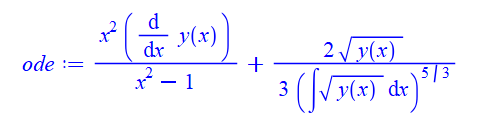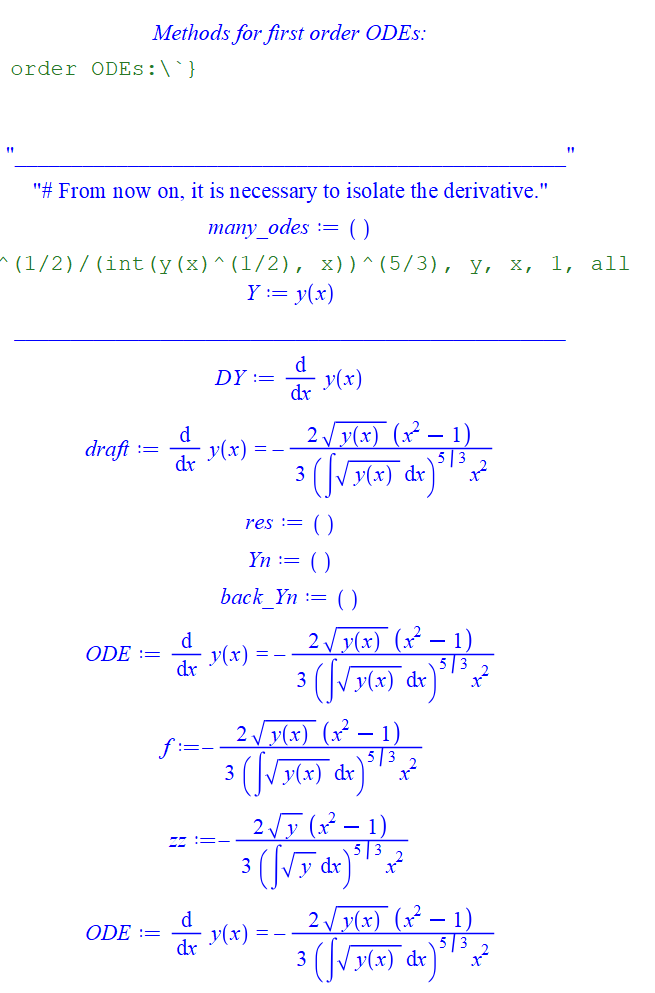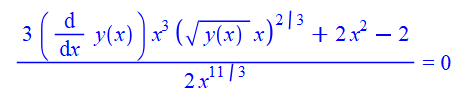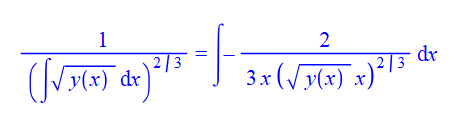4 years, 11 days

## Minerr equivalent to NLPsolve?...

Attached you will find a way in Maple. Since the problem is nonlinear there can be more minima (local or global). That's most likely the reason why the result from Maple and Mathcad do not agree in a first attempt.

NLPsolve (and the Optimization package in general) has a bunch of options Minerr in a solve block does not seem to have. If you want to reproduce whatever Minerr does you have to go through all the options and their combinations. I would start with methods first.(1)(2)(3)

In Mathcad I used solve by using a solve block:But what is the most efficient way to do this in Maple?Supposingin Mathcad is equivalent toin MapleAnd in the same waycould be written using element wise operators(4)(5)(6)(7)(8)(9)

Same as initial points(10)NLPSolve does not give Minerr output as I would have expected from the description

## a subsway...

For comparision:
The best I could do with substitutions. What makes it tricky is the coefficient R1*R2 of s.

I spare out the sorting detail since its already twice as long as acers solution.(1)(2)(3)(4)(5)(6)(7)(8)## General technique...

Your parameters still do not match. In the following I have tried to show how the substitution should be done and what needs to be changed.Original expression(1)

with the following indeterminates(2)

Expressions to substitute (in the form of an equation. Do not use the assignment operator :=     )(3)

Isolate an indeterminate you want to replace(4)

Substitute it into (1)(5)

Now you have Pr appearing which gets you closer to the result you are looking for.

Similarly you have to proceed for the other parameters with  the goal to remove all indeterminates you do not wantfrom your original expression . To get an overview what indeterminates are used in a expression use the indets command.(6)

you get(7)

You can compare which indeterminates are used in the original and the target to determine what has to be removed from the original(8)

The indeterminates above have to be removed from the original and(!) these ones should appear in the target(9)

You have to do this with additional relations, which are your parameter expressions.(10)(11)andThe parameters highlighted in red are neither in the original nor in the target. Substituting your parameter expressions (somehow rearranged -> see isolate above) in the original will introduce them in to the result.
That is what I called mismatch.Further observations:

 • Make clear whether the D inis meant to be the differential operator  or used to name variables or parameters.
 • If the subscripts inare meant to be subscripts and not elements of an indexed object rather use two under scores __ to enter the subscript.
 •(i.e. gamma) inis a constant in Maple that evaluates to a number. It is dangerous to use it as a name.## Collect...

L1 := -L*(k - 1);
collect(L1, L);
(-k + 1) L



preserves a "simplified" factor (sign of your original factor has changed). Collect should also work in more complex cases unless there are other sub-expressions where you don't want to collect L. In those cases changing the sign of the factor like this

Lm := (simplify(k*sqrt(L*L)) assuming (0 < L));
L1 := (factor(-L + Lm) assuming (0 < L and 0 < Lm and 0 <= k));

might work as well.

## Working backward...

Infolevel confirms that dsolve integrates a first order ode

intde := int(x^2*diff(y(x), x)/(x^2 - 1), x) = int(y(x)^(1/2), x)^(-2/3);
infolevel[dsolve] := 5;
dsolve(intde);
Methods for first order ODEs:
--- Trying classification methods ---
trying homogeneous types:
differential order: 1; looking for linear symmetries
trying exact
<- exact successful


If you additionally increase printlevel up to 50 you will find the ODE (which can also be derived by differention of the original equation w.r.t. to x).Edit: In this way Maple reduces the inital equation to a first oder equation.

Then appropriate methods are appliedThis is the first order integroDE Maple integrates. An ODE without integral that integrates to the same result would be this oneTo get there, you have to find a way to convert the right handside of your problem like this (i.e. getting the integration out of the denominator):The attachment shows where the above right hand side comes from.

integrode_backward.mw

## A base like that with a z-axis?Any prefe...

A base like that with a z-axis?